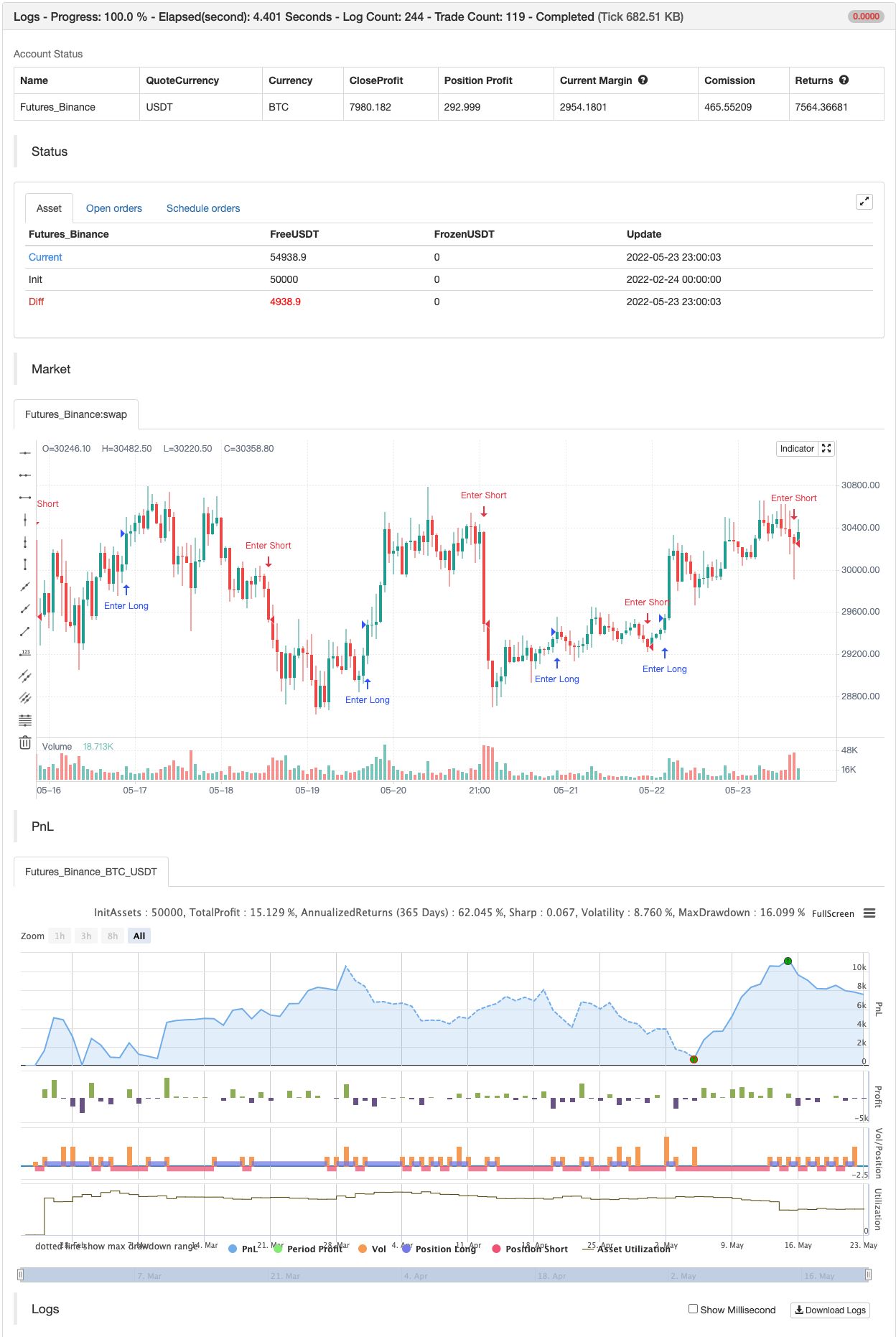# Broken Fractal : Someone's broken dream is your profit!

Author: 张超, Date: 2022-05-25 17:21:02
Tags: bullish bearish

Idea The idea is simple : when market turns around, it traps a bunch of traders off guard. We trade with them, in the same direction of their exit!

Method We let the market first create a fractal We then let the market create an opposite fractal We then let the market break the first fractal it created, thereby trapping lots of trades in the process We then patiently wait till the market gives these trapped traders a chance to exit - and we trade in the same direction

How to use? Green boxes are for long entry, red boxes are for short. Whenever a box appears, that’s the risk criteria - setup limit orders and trade along! Works on all timeframes

If you like this script, please leave a note on how you are using it. I personally use it with Higher Timeframe bias.

PS1 : some traders call this Break of market structure, some call it Breaker, I just call it “Broken Fractal” PS2 : Break of a broken fractal is also very potent. Watch out for those!

backtest```/*backtest
start: 2022-02-24 00:00:00
end: 2022-05-24 00:00:00
period: 1h
basePeriod: 15m
exchanges: [{"eid":"Futures_Binance","currency":"BTC_USDT"}]
*/

// This source code is subject to the terms of the Mozilla Public License 2.0 at https://mozilla.org/MPL/2.0/

//@version=4
study("Broken Fractal", overlay=true)
n = input(title="n==1 or 2", defval=2, type=input.integer)
bgColor = input(title="bgColor", type=input.bool, defval=false)
drawBoxes = input(title="drawBoxes", type=input.bool, defval=true)
showBullishSignal = input(title="showBullishSignal", type=input.bool, defval=true)
showBearishSignal = input(title="showBearishSignal", type=input.bool, defval=true)

var fractalCounter = 0
var highAtDownFractal = 0.0
var lowAtUpFractal = 0.0

downFractal = (n == 2 ? (high[n-2] < high[n]) and (high[n-1] < high[n]) and (high[n+1] < high[n]) and (high[n+2] < high[n]) : (high > high) and (high > high))
// plotchar(downFractal, char='⮝', location=location.abovebar, offset=-1*n, color=color.red, transp=0, title="Down Fractal")
if downFractal
//line.new(x1=bar_index-1, y1=high[n], x2=bar_index, y2=high[n], extend=extend.none, color=color.silver, style=line.style_solid, width=1)
if fractalCounter > 0
fractalCounter := 0
highAtDownFractal := high[n]
fractalCounter := fractalCounter - 1

upFractal = (n == 2 ? (low[n-2] > low[n]) and (low[n-1] > low[n]) and (low[n+1] > low[n]) and (low[n+2] > low[n]) : (low < low) and (low < low))
// plotchar(upFractal, char='⮟', location=location.belowbar, offset=-1*n, color=color.green, transp=0, title="Up Fractal")
if upFractal
//line.new(x1=bar_index-1, y1=low[n], x2=bar_index, y2=low[n], extend=extend.none, color=color.silver, style=line.style_solid, width=1)
if fractalCounter < 0
fractalCounter := 0
lowAtUpFractal := low[n]
fractalCounter := fractalCounter + 1

sellSignal = (fractalCounter < 0) and (open > lowAtUpFractal) and (close < lowAtUpFractal)
//bgcolor(color=(sellSignal and bgColor and showBearishSignal ? color.red : na), transp=80)
//                      if sellSignal and drawBoxes and showBearishSignal
//box.new(left=bar_index, top=lowAtUpFractal, right=bar_index+10, bottom=highAtDownFractal, bgcolor=color.new(color.red, 90), border_color=color.new(color.red, 10))

buySignal = (fractalCounter >= 1) and crossover(close, highAtDownFractal)
//bgcolor(color=(buySignal and bgColor and showBullishSignal ? color.green : na), transp=80)
//if buySignal and drawBoxes and showBullishSignal
//box.new(left=bar_index, top=highAtDownFractal, right=bar_index+10, bottom=lowAtUpFractal, bgcolor=color.new(color.green, 90), border_color=color.new(color.green, 10))